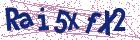# On convergence and stability conditions of homotopy perturbation method for an inverse heat conduction problem

Document Type : Research Article

Authors

Faculty of Mathematics, K. N. Toosi University of Technology, Tehran, Iran.

Abstract

In this paper, we investigate the application of the Homotopy Perturbation Method (HPM) for solving a one-dimensional nonlinear inverse heat conduction problem. In this problem the thermal conductivity term is a linear function with respect to unknown heat temperature in bounded interval. Furthermore, the temperature histories are unknown at the end point of the interval. This problem is ill-posed. So, using the finite difference scheme and discretizing the time interval, the partial differential equation is reduced into a System of Nonlinear Ordinary Differential Equations (SNODE's). Then, using HPM, the approximated solution of the obtained Ordinary Differential Equation (ODE) system is determined. In the sequel, the stability andconvergence conditions of the proposed method are investigated. Finally, anupper bound of the error is provided.

Keywords20.1001.1.24236977.2015.5.2.6.3

#### References

1. Adomian, G. A new approach to the heat equation-An application of the decomposition method, J. Math. Anal. Appl. 113 (1986) 202-209.
2. Alifonov, O.M. Inverse heat transfer problems, Springer-Verlag, 1994.
3. Beck, J.V., Blackwell, B. and St. Clair. Ch. J. Inverse heat conduction, ill-posed problems, John Willey and Sons, Inc, 1985.
4. Buhmann Martin, D. Radial Basis Functions: Theory and Implementations, Cambridge University Press, 2003.
5. Cannon, J.R. The one dimensional heat equation, Addison-Welsey , New york, 1984.
6. Dehghan, M. and Shakeri, F. Solution of a partial differential equation subject to temperature oversimplification by He's homotopy perturbation method, Phys. Scr., 75 (2007) 778-787.
7. Douglas, J.J. and Russell, T.F. Numerical Methods for Convection Dominated Diffusion Problems Based on Combining the Method of Characteristics with Finite Element or Finite Difference Procedures, SIAM J.
Numer. Anal., 19 (5) (2006) 871-885
8. Ghasemi, M., Tavassoli Khanjani, M. and Davari, A. Numerical solution of two -dimensional nonlinear differential equation by homotopy perturbation method, Appl. Math. Comput. 189 (2007) 341-345.
9. He, J. H. Some asymptotic methods for strongly nonlinear equations, International Journal of Modern Physics B, 20 (2006) 1141-1199.
10. He, J. H. An elementary introduction to recently developed asymptotic methods and nanomechanics in textile engineering, International Journal of Modern Physics B, 22 (2008) 3487-3578.
11. He, J. H. Homotopy perturbation technique, Comput. Methods. Appl. Mech. Engrg., 178 (1999) 257-262.
12. He, J. H. A coupling method of a homotopy technique and a perturbation technique for non-linear problems, Int. J. Non-Linear Mechanics, 35 (2000) 37-43.
13. He, J. H. The homotopy perturbation method for nonlinear oscilators with discontinuities, Appl. Math. Comput., 5 (2006) 287-292.
14. He, J. H. Homotopy perturbation method for solving boundary value problems, Phys. lett. A., 35 (2006) 87-88.
15. Johansson, B.T. and Lesnic. D. A method of fundamental solutions for transient heat conduction, Engineering Analysis with Boundary Elements, 32 (2008) 697-703.
16. Jia, H., Xu, W., Zhao, X. and Li, Z. Separation of variables and exact solutions to nonlinear diffusion equations with x f dependent convection and absorption, J. Math. Anal. Appl., 339 (2008) 982-995.
17. Johansson, B.T. and Lesnic, D. Determination of spacewise depedent heat suorce, Journal of computational and Applied Mathematics, 209 (2007)66-88.
18. Lewandowski, J.L.V. Marker method for the solution of nonlinear diffusion equations, JCAM., 196 (2006) 523-539.
19. Onyango, T.T.M., Ingham, D.B., Lesnic, D. and Soldicka, M. Determination of a time-dependent heat transfer coefficient from non-standard boundary measurements, Mathematics and Computers in Simulation, 79
(2009) 1577-1548
20. Qu, C., Ji, L. and Dou, J. Exact solution and generalized conditional symmetries to (n + 1)ff dimensional nonlinear diffusion equations with source term, Physics Letters A, 343 (2005) 139-147.
21. Shidfar, A. and Zakeri, A. A numerical method for backward inverse heat conduction problem with two unknown functions, International Journal of Engineering science, 16 (2008) 71-74.
22. Shidfar, A. and Zakeri, A. Asymptotic solution for an inverse parabolic problem, Mathematica Balkanica, 18 (2004) 475-483.
23. Shidfar, A. and Zakeri, A. A two-dimensional inverse heat conduction problem for estimating heat source, International Journal of Applied Mathematics and Mathematical Science, 10 (2005) 1633-1641.
24. Siddiqui, A.M., Mahmood, R. and Ghori, Q.K. Homotopy perturbation method for thin film of a fourth gradeuid down a vertical cylinder, Phys. lett. A., 352 (2006) 404-410.
25. Vogel, C.R. Computational methods for inverse problems, SIAM, 2002.
26. Zakeri, A., Aminataei, A. and Jannati, Q. Application of He's homotopy perturbation method for Cauchy problem of ill-posed nonlinear diffusion equation, Discrete Dynamics in Nature and Society, Vol. 2010, ID 780207.
27. Zakeri, A. and Jannati, Q. An inverse problem for parabolic partial differential equations with nonlinear conductivity term in one dimensional space, Scholarly Research Exchange, 2009, ID 468570.
28. Zakeri, A. and Jannati, Q. Stability of homotopy perturbtion technique for an inverse diffusion problem, The proceeding of fourty fourth Annual Iranian Mathematics Conference, pp. 682-685, Rafsanjan, Aug . 2014.